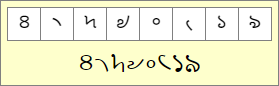## Monday 31 January 2022

### Unicode Trivia U+09F8

Codepoint: U+09F8 "BENGALI CURRENCY NUMERATOR ONE LESS THAN THE DENOMINATOR"
Block: U+0980..09FF "Bengali"

Decimal Day. 15 February 1971. A Monday. The day the United Kingdom and the Republic of Ireland converted to decimal currency. Before that, each pound was divided into twenty shillings and each shilling into twelve pence. We'll ignore farthings.

So, if I bought something worth one penny with an old, pre-decimalisation five pound note, I'd get a dirty look and the following change:

£4 19/11 = £4. 19s. 11d = 4 pounds, 19 shillings, 11 pence

Such mixed-radix currencies were not uncommon. In British India, the rupee had been divided into sixteen annas, each anna into four pice (paisa), and each pice into three pies. The change from five rupees for a one pie item would be:

Rs. 4/15/3/2 = 4 rupees, 15 annas, 3 pice, 2 pies

In pre-decimal Bengal, the taka (rupee) had been divided into sixteen ana, and each ana into twenty ganda. The change from five taka for a one ganda item would be:

Tk. 4/15/19 = 4 taka, 15 ana, 19 ganda

Of course, that's in English using the Latin script and Western Arabic numerals. In Bengali, one could have written:

৪৲৸৶৹৻১৯U+09EA U+09F2 U+09F8 U+09F6 U+09F9 U+09FB U+09E7 U+09EF

As Anshuman Pandey, points out, only one currency mark was actually used when multiple units were written. We'll return to this in due course, but in the meantime I've left that refinement out of the example above.

Bengali is a Brahmic script written left-to-right, so in Unicode this example is:

1. U+09EA "BENGALI DIGIT FOUR"
2. U+09F2 "BENGALI RUPEE MARK"
3. U+09F8 "BENGALI CURRENCY NUMERATOR ONE LESS THAN THE DENOMINATOR"
4. U+09F6 "BENGALI CURRENCY NUMERATOR THREE"
5. U+09F9 "BENGALI CURRENCY DENOMINATOR SIXTEEN"
6. U+09FB "BENGALI GANDA MARK"
7. U+09E7 "BENGALI DIGIT ONE"
8. U+09EF "BENGALI DIGIT NINE"

The first two glyphs ("৪৲") represent "4 taka" in decimal; the Bengali digit four just happens to look like a Western Arabic digit eight. The next three glyphs ("৸৶৹") represent "15 ana". This is complicated by the fact that, traditionally, ana were written as fractions of a taka. Finally, the last three glyphs ("৻১৯") represent "19 ganda" in decimal where, just to confuse us further, the ganda mark comes before the digits, not after them as with taka and ana.

The ana component is the most perplexing. The Unicode codepoint name for U+09F8, "BENGALI CURRENCY NUMERATOR ONE LESS THAN THE DENOMINATOR", doesn't really help. Fortunately, there's an explanation within the much later proposal to add the ganda mark in 2007.

The fifteen possible quantities of ana are:

• ৴৹ = 1 ana (Numerator 1)
• ৵৹ = 2 ana (Numerator 2)
• ৶৹ = 3 ana (Numerator 3)
• ৷৹ = 4 ana (Numerator 4)
• ৷৴৹ = 5 ana
• ৷৵৹ = 6 ana
• ৷৶৹ = 7 ana
• ৷৷৹ = 8 ana
• ৷৷৴৹ = 9 ana
• ৷৷৵৹ = 10 ana
• ৷৷৶৹ = 11 ana
• ৸৹ = 12 ana (Numerator One Less Than the Denominator)
• ৸৴৹ = 13 ana
• ৸৵৹ = 14 ana
• ৸৶৹ = 15 ana

This looks like a modified base-4 tally mark system. But, thinking back to what Anshuman Pandey said about elided currency marks, I wonder if this scheme didn't originate in a finer-grained positional system.

Imagine that instead of the taka being divided directly into sixteen ana, it was divided into four virtual "beta", which were themselves divided into four virtual "alpha". Obviously:

• ana = alpha + beta * 4

But now we have the following encoding:

• ৴ = 1 alpha (Numerator 1)
• ৵ = 2 alpha (Numerator 2)
• ৶ = 3 alpha (Numerator 3)
• ৷ = 1 beta
• ৷৷ = 2 beta
• ৸ = 3 beta (Numerator One Less Than the Denominator)

For beta, the "denominator" is indeed four, to the mysterious U+09F8 "BENGALI CURRENCY NUMERATOR ONE LESS THAN THE DENOMINATOR" suddenly makes sense.

We can now come up with an algorithm for writing out a currency amount according to the scheme described by Anshuman Pandey:

• Let T, A, G be the number of taka (0 or more), ana (0 to 15), ganda (0 to 19) respectively
• If T is not zero then
• Write out the Bengali decimal representation of T
• If both A and G are zero
• Write out U+09F2 "BENGALI RUPEE MARK"
• We're finished
• Let α be A modulo 4 (0 to 3)
• Let β be A divided by 4, rounded down (0 to 3)
• If β is 1, write out U+09F7 "BENGALI CURRENCY NUMERATOR FOUR"
• If β is 2, write out U+09F7 "BENGALI CURRENCY NUMERATOR FOUR" twice
• If β is 3, write out U+09F8 "BENGALI CURRENCY NUMERATOR ONE LESS THAN THE DENOMINATOR"
• If α is 1, write out U+09F4 "BENGALI CURRENCY NUMERATOR ONE"
• If α is 2, write out U+09F5 "BENGALI CURRENCY NUMERATOR TWO"
• If α is 3, write out U+09F6 "BENGALI CURRENCY NUMERATOR THREE"
• If G is zero then
• Write out U+09F9 "BENGALI CURRENCY DENOMINATOR SIXTEEN"
• We're finished
• Write out U+09FB "BENGALI GANDA MARK"
• Write out the Bengali decimal representation of G
• We're finished

For our example, T=4, A=15, G=19, α=3, β=3 and the output is:

৪৸৶৻১৯

U+09EA U+09F8 U+09F6 U+09FB U+09E7 U+09EF

This representation is surprisingly concise and totally unambiguous.